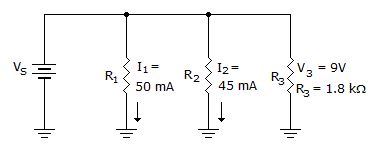# Electronics - Parallel Circuits

Exercise : Parallel Circuits - General Questions
31.
A parallel circuit is also used as a divider for:
conductance
voltage
power
current
Explanation:
No answer description is available. Let's discuss.

32.
What is RT for a 12 k, a 4 k, and a 3 kresistor in parallel?
1.5 k2 k6.3 k19 kExplanation:
No answer description is available. Let's discuss.

33.
What is the total resistance of four 1 kparallel-connected resistors?
200 ohms
250 ohms
400 ohms
4 kilohms
Explanation:
No answer description is available. Let's discuss.

34.
Which are the better test devices used to isolate a suspected open component within a parallel circuit?
a voltmeter or an ohmmeter
neither an ammeter nor a voltmeter
a wattmeter or a voltmeter
an ammeter or an ohmmeter
Explanation:
No answer description is available. Let's discuss.

35.What does P2 equal in the given circuit?

45 mW
405 mW
450 mW
900 mW